Latest Banking jobs   »   Reasoning Ability Quiz For IDBI AM/Executive...

# Reasoning Ability Quiz For IDBI AM/Executive 2022- 5th June

Directions (1-5): Study the following information carefully and answer the questions given below-
Eight persons P, Q, R, S, T, U, V, W go for a vacation in different months of a year viz. March, April, May, June, July, August, September, and October. S goes in a month which has 31 days. Three persons go for vacation in between R and S. P goes for a vacation immediately before T but not in a month which has 31 days. Only two persons go for vacation in between W and Q. T goes in a month before S. Only one person goes in between U and V. W goes before S. U does not go after Q.

Q1. Who among the following go for vacation in May?
(a) P
(b) S
(c) R
(d) T
(e) None of these

Q2. R goes for a vacation in which of the following month?
(a) August
(b) March
(c) June
(d) September
(e) None of these

Q3. How many persons go for vacation in between W and V?
(a) One
(b) Two
(c) Three
(d) Four
(e) None of the above

Q4. Four of the following are alike in a certain way so form a group, who among the following does not belong to that group?
(a) V
(b) S
(c) R
(d) T
(e) Q

Q5. Who among the following go for vacation in June?
(a) P
(b) U
(c) W
(d) T
(e) None of these

Directions (6-10): In each of the questions below are given Some statements followed by Some Conclusions. You have to take the given statements to be true even if they seem to be at variance from commonly known facts. Read all the statements and then decide which of the given Conclusions logically follows from the given statements disregarding commonly known facts.

Q6.Statements:
All Chocolates are Toffee.
Some Toffee are Lollipop.
Some Candy are Lollipop.

Conclusions:
I. Some Toffee are Chocolates.
II. All Lollipop are Chocolates is a possibility.
III. Some Lollipop are not toffee.
(a) Only I follows
(b) Only II follows
(c) Only I and II follow
(d) Only III follows
(e) None follows

Q7. Statements:
Some News are Impact.
Some Impact are Reactions.
All Reactions are Important.

Conclusions:
I. Some Important are News is a possibility.
II. Some Important can be Impact.
III. All Reactions are not Impact.
(a) Only II follows
(b) Only II and III follow
(c) Only I and III follow
(d) Only I and II follow
(e) None of these

Q8. Statements:
All Great are Achievement.
All Achievement are Stable.
All Focus are Stable.

Conclusions:
I. Some Achievements can be Focus.
II. Some Stable are not Great.
III. All Great are Stable.
(a) Only I follows
(b) Only II follows
(c) Only III follows
(d) Only I and III follow
(e) None of these

Q9. Statements:
Some Nice are Good.
All Good are Fine.
All Fine are Excellent.

Conclusions:
I. Some Nice are Excellent.
II. Some Fine are not Good.
III. No Fine are Nice is a possibility.
(a) Only I follows
(b) Only II follows
(c) Only III follows
(d) Only I and II follow
(e) None of these

Q10. Statements:
Some Straight are Line.
Some Straight are Diagonal.
Some Divide are Line.

Conclusions:
I. Some Straight can be Divide.
II. Some Straight are not Diagonal.
III. Some Straight are Divide.
(a) Only I and III follow
(b) Only I follows
(c) Either I or III follow
(d) Only III follows
(e) None of these

Directions (11-14): Each of these questions is based on the following information:
(i) A % B means A is the daughter of B.
(ii) A @ B means A is the mother of B.
(iii) A \$ B means A is the father of B.
(iv) A * B means A is the son of B.
(v) A © B means A is brother of B

Q11. Which of the following shows the relation that S is sister-in-law of Q?
(a) S @ T * A © R % P \$ Q
(b) S % A @ T * R © Q \$ P
(c) T @ S © R \$ Q % P
(d) R % S \$ P * Q
(e) None of these

Q12. Who among the following is F’s niece in this relation- ‘A \$ D @ G % E © F’?
(a) A
(b) D
(c) G
(d) E
(e) Can’t be determine

Q13. If the expression ‘P % R \$ S © T @ W’ true, then which of the following is true?
(a) P is brother of T
(b) S is daughter of R
(c) P is aunt of W
(d) R is grandmother of W
(e) None is true

Q14. If the expression ‘A * D @ P % Q © E \$ R’ true, then which of the following is false?
(a) E is uncle of A
(b) P is daughter of D
(c) Q is aunt of R
(d) E is brother-in-law of D
(e) All are true

Q15. Showing a man on the stage, Rita said, ” He is the brother of the daughter of the wife of my husband. How is the man on stage related to Rita?
(a) Son
(b) Husband
(c) Cousin
(d) Nephew
(e) Niece

Solutions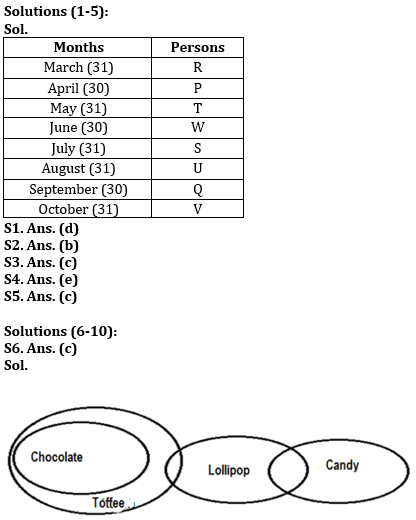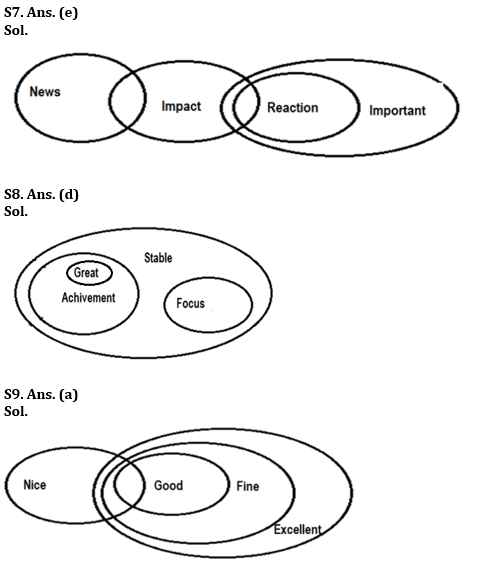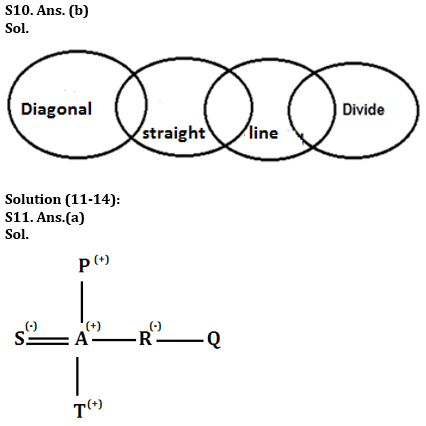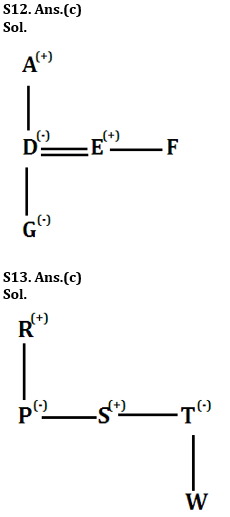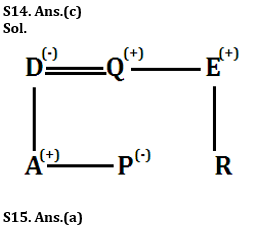#### Congratulations!Incorrect details? Fill the form again here

•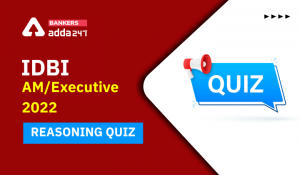Reasoning Ability Quiz For IDBI AM/Execu...
•Reasoning Ability Quiz For IBPS RRB PO P...
•Important Current Affairs Quiz for Bank ...
•Reasoning Ability Quiz For IDBI AM/Execu...
•Quantitative Aptitude Quiz For IDBI AM/E...
•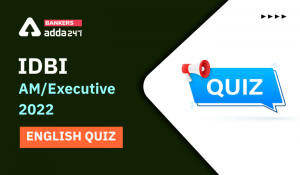English Quizzes For IDBI AM/Executive 20...